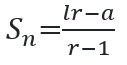# Geometric SeriesIntroduction to Sequence and Series
Arithmetic series
Some of the step in the note below are skipped so check the notes above as reference for ease
Geometric Sequence:

→The sequence whose common ratio between the two successive terms are same is called geometric sequence.
For example:3, 9, 27, 81……..

General term of Geometric Progression is given by;

tn=arn-1

where,
tn= nth term of sequence
a  = first term of sequence
n  = nth term of sequence
r  = common ratio of sequence

Geometric Mean: The term/s in between the first and last term in the arithmetic progression is/are called geometric means.
Example; i) 2, 4, 8                   ii) 4, -8, 16, -32, 64
Here, the highlighted terms are the geometric means.

Formulas related to Geometric Means:

The single geometric mean between two terms a & b is calculated by;If there are n terms between ‘a’ and ‘b’ then the common ratio is given by;Here, n does represent number of terms in the sequence but number of means between first and last term.

nth mean=arn

Sum of GP[when 1st term and number of terms are given & r >1]

﻿→[when 1st term and number of terms are given & r <1][when 1st and last terms are given & r >1][when 1st and last terms are given & r <1]